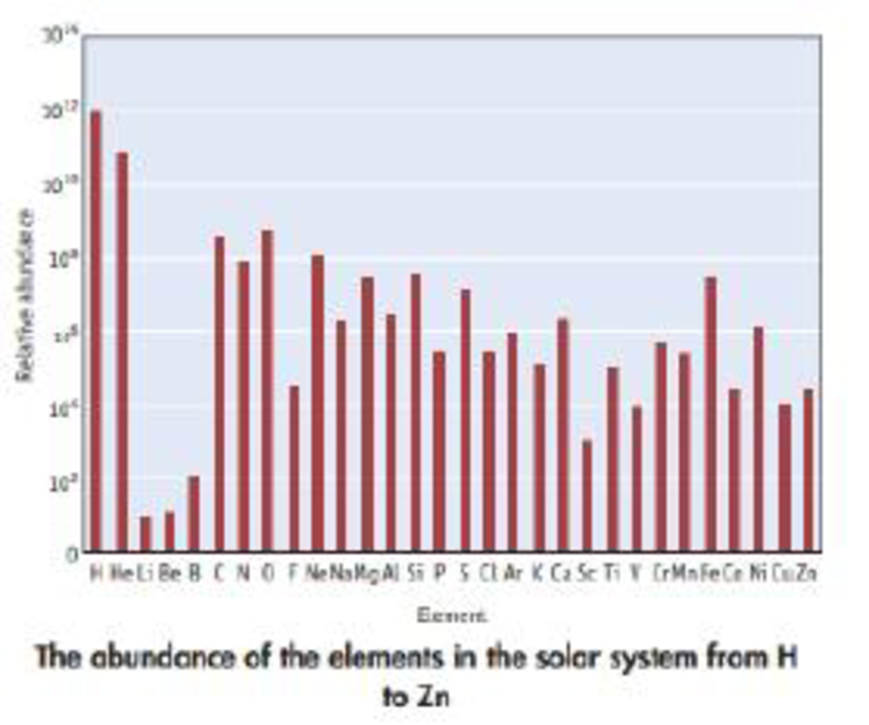Chapter 2, Problem 104GQ

Chapter
Section
Textbook Problem

The following chart shows a general decline in abundance with increasing mass among the first 30 elements. The decline continues beyond zinc. Notice that the scale on the vertical axis is logarithmic, that is, it progresses in powers of 10. The abundance of nitrogen, for example, is 1/10,000 (1/104) of the abundance of hydrogen. All abundances are plotted as the number of atoms per 102 atoms of H. (The fact that the abundances of Li, Be, and B, as well as those of the elements near Fe, do not follow the general decline is a consequence of the way that elements are synthesized in stars.)(a) What is the most abundant main group metal? (b) What is the most abundant nonmetal? (c) What is the most abundant metalloid? (d) Which of the transition elements is most abundant? (e) Which halogens are included on this plot, and which is the most abundant?

(a)

Interpretation Introduction

Interpretation: For the given conditions, the elements are needed to be identified.

Concept introduction:

Main group elements are included from group 1A to 8A .

Nonmetals: the right of diagonal elements stretches from boron to Tellurium, in group 14 to 18.

Metalloids: the elements in between metals and nonmetals or the diagonal elements stretch from boron to Tellurium.

Transition elements are included in 1B to 8B group’s elements.

Halogen is the elements included in group 7A.

Explanation

In the given chart, Li, Be, Mg, Al, K and Ca are the main group metals present

(b)

Interpretation Introduction

Interpretation: For the given conditions, the elements are needed to be identified.

Concept introduction:

Main group elements are included from group 1A to 8A .

Nonmetals: the right of diagonal elements stretches from boron to Tellurium, in group 14 to 18.

Metalloids: the elements in between metals and nonmetals or the diagonal elements stretch from boron to Tellurium.

Transition elements are included in 1B to 8B group’s elements.

Halogen is the elements included in group 7A.

(c)

Interpretation Introduction

Interpretation: For the given conditions, the elements are needed to be identified.

Concept introduction:

Main group elements are included from group 1A to 8A .

Nonmetals: the right of diagonal elements stretches from boron to Tellurium, in group 14 to 18.

Metalloids: the elements in between metals and nonmetals or the diagonal elements stretch from boron to Tellurium.

Transition elements are included in 1B to 8B group’s elements.

Halogen is the elements included in group 7A.

(d)

Interpretation Introduction

Interpretation: For the given condition, the elements are needed to be identified.

Concept introduction:

Main group elements are included from group 1A to 8A .

Nonmetals: the right of diagonal elements stretches from boron to Tellurium, in group 14 to 18.

Metalloids: the elements in between metals and nonmetals or the diagonal elements stretch from boron to Tellurium.

Transition elements are included in 1B to 8B group’s elements.

Halogen is the elements included in group 7A.

(e)

Interpretation Introduction

Interpretation: For the given conditions, the elements are needed to be identified.

Concept introduction:

Main group elements are included from group 1A to 8A .

Nonmetals: the right of diagonal elements stretches from boron to Tellurium, in group 14 to 18.

Metalloids: the elements in between metals and nonmetals or the diagonal elements stretch from boron to Tellurium.

Transition elements are included in 1B to 8B group’s elements.

Halogen is the elements included in group 7A.

Still sussing out bartleby?

Check out a sample textbook solution.

See a sample solution

The Solution to Your Study Problems

Bartleby provides explanations to thousands of textbook problems written by our experts, many with advanced degrees!

Get Started

Why is the ion not found in nature?

Chemistry for Engineering Students

Problem 17-3 Write the IUPAC name for each compound.

Introduction to General, Organic and Biochemistry

What is population genetics?

Human Heredity: Principles and Issues (MindTap Course List)